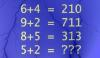BRAIN TEASERSBrain Teasers User Profile

 Mathematical Puzzle: IF 6+4=... Mathematical Puzzle: IF 6+4=210, 9+2=711 and 8+5=313 THEN 5+2=?